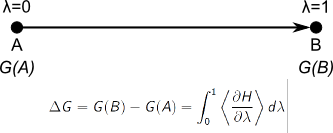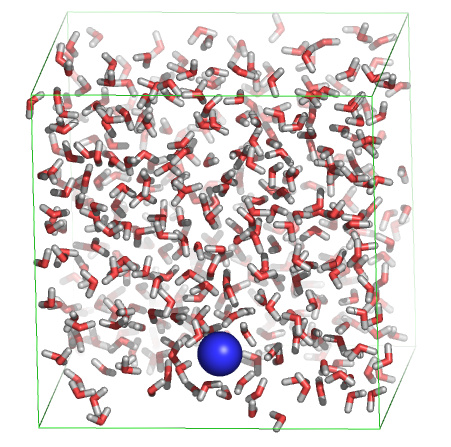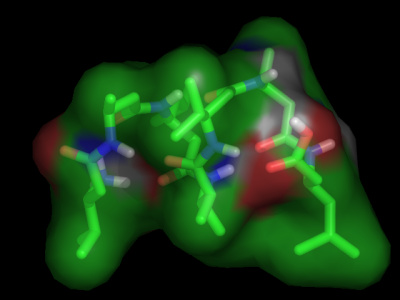# Practical 4: Free energy calculations

#### Bert de Groot

Contents
The free energy "slow-growth" method
Solvation free energy of a sodium ion in water
Thermodynamics of reversible peptide folding
References

## The free energy "slow-growth" method

Molecular simulations can be carried out to gain either dynamical or energetical information on the simulated system. Today we'll focus on different ways to extract energies and free energies from simulations. One way to gain access to free energies by simulation is to perform so-called free-energy perturbation (FEP) simulations. In such simulations, the system is gradually "morphed" from one state to another (let's say from state "A" to state "B"), during which the effect of this perturbation onto the free energy is monitored. In practice, this is achieved by defining a morph parameter lambda, which is defined to be zero in the initial state (A), and one in the final state (B). By collecting the derivative of the potential energy with respect to lambda during the simulation, and by integrating over lambda afterwards, we gain access to the free energy.Go back to Contents

## Solvation free energy of a sodium ion in waterWe are now going to use this procedure to calculate the free energy of solvation for a sodium ion in water. For this, we'll slowly create a sodium ion in a water box. In order to start the simulation, download the starting coordinates, the topology, and the MD parameter file first. First have a look at the starting structure:

```rasmol start.pdb
```

In rasmol, type:

``` wireframe 50
unitcell true
select na
cpk
```

Note that the sodium ion is already "present" in the starting structure. Now have a look at the topology "na.top", with 'xedit', 'nedit', 'more' or 'less', and look for the line containing "Na" under "[atoms]". You'll notice that two states (A and B) are defined, with charge 0 and 1, respectively. This means that during the simulation, the charge of the sodium ion will be slowly switched on.
Now start the simulation:

``` gmx grompp -f fep -c start.pdb -p na -maxwarn 1
gmx mdrun -v -c charged.gro
```

Analyse the free energy change by:

``` xmgrace dhdl.xvg
```

In order to compute the approximate free energy, we must integrate dH/dlambda over dlambda. Instead, we have dH/dlambda as a function of time. This is not a problem, as we know that lambda changed from0 to 1 during this time (10 ps). So instead, we can integrate the curve over time, and divide the obtained value by ten to derive the desired free energy. To integrate, under "Data", select "Transformations", followed by "Integration", and press "Accept".

Question: The experimentally observed solvation free energies for sodium range from -365 to -372 kJ/mol. How does the obtained value correspond to that?

Instead of integrating dH/dlambda, we can also compare the initial and final values of the potential energy of the system:

```gmx energy
```

Select "Potential", followed by "0" (and, if necessary, an extra "enter").

```xmgrace energy.xvg
```

Subtract the initial value from the final value.

Question: What value do you get? Why is this value different from than the free energy difference obtained by integrating dH/dlambda?

One important check in simulations in general and in free energy simulations in particular is to make sure that the answer has converged. In order to do so, we can either perform a longer (or shorter!) simulation, and compare the result to the original, or we can perform the backward transition to see if the free energy difference is the opposite of the forward transition. In this practical, we'll do both. First, we'll carry out the backward transition. Copy the MD parameter file to incorporate the changes:

```cp fep.mdp back.mdp
```

and open "back.mdp" in your favorite editor (xedit, nedit, emacs, kate, vi) to make the following changes:
First search for "Free energy", then change "init-lambda" to 1 and change delta-lambda to "-0.0002". Now we can start the backward simulation. For this, we'll use the final structure of the previous simulation (charged.gro) as initial structure:

```gmx grompp -f back -c charged.gro -p na -maxwarn 1
gmx mdrun -v
```

And analyse the free energy change using xmgrace. Remember that we now changed lambda from one to zero, meaning that if we integrate over time, we should divide the obtained value by -10 instead of by 10.

Question: What value do you get? How does this value compare to the free energy change for the forward simulation? Would you consider the obtained value for the solvation free energy sufficiently converged?

Question: If we take the forward and backward simulation together, is the total free energy change positive or negative? Which of the two should it have been?

As stated above, another way to check convergence is to perform a longer simulation and see if the free energy change remains the same as compared to a shorter simulation. For this, open "fep.mdp" in your favorite editor. Change "nsteps" from 5000 to 50000, and "delta-lambda" from 0.0002 to 0.00002, and repeat the forward transition:

```gmx grompp -f fep -c start.pdb -p na -maxwarn 1
gmx mdrun -v
```

Question: Is the obtained free energy very different as compared to the shorter simulations? What would you consider an appropriate length for this type of simulation?

Go back to Contents

## Thermodynamics of reversible peptide foldingFor the case of a sodium ion in water we could compute the free energy difference between two states because we defined a path connecting the two states, and we had access to the free energy gradient along the path. In the case of the ion in water, the path was an artificial path (hence such free energy perturbation studies are also called "computational alchemy"). Likewise, a free energy difference can also be computed along a real path, e.g. by forcing an ion across an electrostatic gradient and integrating the force required to displace the ion along the path.
There are also cases, however, where such a path, artificial or real, can not be easily defined. Peptide or protein folding is a good example. The problem of defining a path is that there are multiple folding (and unfolding) pathways that connect the (well-defined) folded state and the degenerate unfolded state. If we would like to compute the folding free energy by integrating the force along a pathway connecting the two, we'd therefore have to follow multiple pathways, and take a weighted average of the results. In the case, however, where we have a simulation in which folding and unfolding takes place spontaneously (i.e. without forcing the system to fold or unfold) and reversibly we can also access the free energy difference between the folded and unfolded state in a different way, namely simply by monitoring the fraction of time the system resides in each state. In the equilibrium case (i.e. if the simulation was long enough to visit all relevant configurations multiple times) the probability of finding the system is exactly proportional to its Boltzmann factor. Therefore, for any two states, the following equation holds:

with
##### DeltaG = G1 - G2

Therefore, if we know the respective probability to find the system in a particular pair of states, we can calculate the free energy difference between these two states. Note that for this type of free energy calculation we solely need the conformational information (coordinates, trajectory) from the simulation, no energies!

To illustrate the above, we are going to calculate the free energy of the folding of a small peptide. For this, download a PDB file of the peptide and the MD trajectories at four different temperatures: 298K, 340K, 350K and 360K.
First view part of one trajectory with VMD:

```gmx trjconv -s pep.pdb -f 340.xtc -o movie.pdb -e 10000 -fit rot+trans
```

and select "4" followed by "1" when asked to select a group.

```vmd movie.pdb
```

in VMD, play with different representations (under Graphics -> Representations, e.g. "Licorice") and press the "play" button on the bottom of the main window. You see the first folding transition during the simulation at 340 K.

Question: What kind of structure is the folded structure?

For a more quantitative analysis of the folding/unfolding transitions, we'll analyse the root mean square deviation of each structure in each trajectory with respect to the folded structure. Here we will call the structure "folded" if it has a root mean square deviation (RMSD) of less than 2 Angstroms (0.2 nm) of the backbone atoms with respect to the reference structure in pep.pdb.

```gmx rms -s pep.pdb -f 340.xtc -o rmsd_340.xvg
```

when asked for a selection of groups, press "4" (Backbone) and "4" (Backbone) again. View the result with xmgrace:

```xmgrace rmsd_340.xvg
```

Question: How many folding/unfolding transitions do you observe?

Repeat the same procedure for the other 3 temperatures to generate rmsd_298.xvg, rmsd_350.xvg and rmsd_360.xvg. With a command like the following, we can count how many configuration from each trajectory are "folded" or "unfolded":

```cat rmsd_340.xvg | awk 'NR >18 &&\$2 < 0.2 {print \$0}' | wc -l
```

if the counting didn't work, then try:

```cat rmsd_340.xvg | sed 's/0\./0,/g' | awk ' NR > 18 && \$2 < 0.2 {print \$0}' | wc -l
```

(never mind if you didn't understand that command completely. We first throw away the header of the rmsd_340.xvg file, and afterwards "awk" only prints the lines of which the second column (the RMSD) is smaller than 0.2; the "wc -l" simply counts the number of lines). The sed command, finally, replaces the dots in the file with comma's, for the case your computer has expects german input, which uses comma's for floating point numbers. The obtained number is the number of "folded" configurations in the 340K simulation. Repeat the procedure, now to count the number of "unfolded" conformations. You'll see, at this temperature the system is folded about one third of the time.

Question: What is the difference in free energy between the folded and unfolded state at this temperature? (Hint: kT = 2.5 kJ/mol at 300K)

Question: What about the other three temperatures? What would you estimate to be the folding temperature?

Question: Do the above free energy estimates, based on peptide configurations, include the influence of the solvent? Would different folding free energies (and/or an estimate of the folding temperature) be obtained when the solvent would be analysed as well?

Question: Assuming that the folding enthalpy is the same for the different temperatures, how can the entropy difference between the folded and unfolded state be estimated from the set of free energies? How large do you estimate the entropy of folding in this case? Is it positive or negative? Why?

Go back to Contents

## Further references

Books:

• Editors: Chipot C. , Christophe A. et al., Free Energy Calculations, Springer Series in Chemical Physics, Vol. 86, [link]